Open in App
Not now

# PHP – MYSQL : sum() operation

• Last Updated : 01 Oct, 2021

Problem Statement:

In this article, we are going to perform sum() aggregate operation on our database using PHP with xampp server.

So we are considering the food_order database and perform database sum() operation.

Requirements:

xampp

Introduction:

PHP stands for hypertext preprocessor which is a server-side scripting language connect with databases.

it is connected with MySQL to perform manipulations in a database.

MySQL is a database query language for managing databases.

• sum()

sum() function is an aggregation operation used to add the particular column based on the given condition.

Syntax:

```SELECT SUM(column1),column2,...column1 from
table_name;```

It can be also used with GROUP BY & HAVING clause.

Example query:

Consider the table: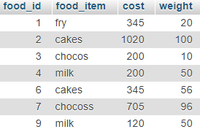Find the total cost of food items

`SELECT  SUM(cost) from food;`

Output:

`Total cost: 2935`

Find the total weight of food items

`SELECT  SUM(weight) from food;`
`Total weight: 382`

Find the total cost of food items with respect to items

```SELECT food_item, SUM(cost) FROM food GROUP BY food_item;
Total cakes = 1365
Total chocos = 200
Total chocoss = 705
Total fry = 345
Total milk = 320```

Approach

• Create  a database
• Create a table
• PHP code to perform sum() operation

Steps

• Start xampp server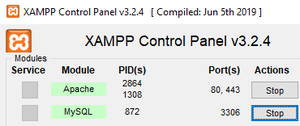• Create a database named geek and create a table named food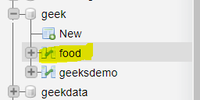Table structure: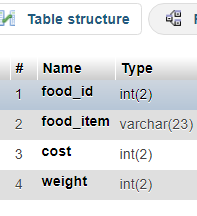Refer this for how to insert records in xampp

https://www.geeksforgeeks.org/performing-database-operations-in-xampp/

Finally, the food table contains• PHP code to perform sum function

form.php

After writing code type “localhost/form.php” to view the output in the browser.

## PHP

 `` `` `"``; ``echo` `"sum() aggregate  Demo "``; ``echo``""``;` `echo` `"

"``;``echo` `" Find the total cost of food items "``;``echo` `"

"``;` `//sql query` `\$sql` `= ``"SELECT  SUM(cost) from food"``;` `\$result` `= ``\$conn``->query(``\$sql``);` `//display data on web page` `while``(``\$row` `= mysqli_fetch_array(``\$result``)){` `    ``echo` `" Total cost: "``. ``\$row``[``'SUM(cost)'``];` `    ``echo` `"
"``;` `}`   `echo` `"

"``;``echo` `" Find the total weight of food items "``;``echo` `"

"``;` `//sql query` `\$sql` `= ``"SELECT  SUM(weight) from food"``;` `\$result` `= ``\$conn``->query(``\$sql``);` `//display data on web page` `while``(``\$row` `= mysqli_fetch_array(``\$result``)){` `    ``echo` `" Total weight: "``. ``\$row``[``'SUM(weight)'``];` `    ``echo` `"
"``;` `}`     `//close the connection`   `\$conn``->close();` `?>` `` ``

Output: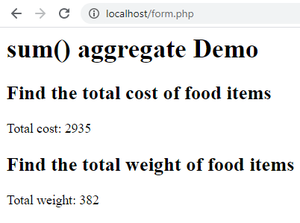Example: sum() with group by clause

form1.php

## PHP

 `` `` `"``; ``echo` `"sum() aggregate with group by Demo "``; ``echo``""``;` `echo` `"

"``;``echo` `" ` ` ``";echo "``

";` `//sql query` `\$sql` `= ``"SELECT food_item, SUM(cost) FROM food GROUP BY food_item"``;` `\$result` `= ``\$conn``->query(``\$sql``);` `//display data on web page` `while``(``\$row` `= mysqli_fetch_array(``\$result``)){` `    ``echo` `"Total "``. ``\$row``[``'food_item'``]. ``" = "``. ``\$row``[``'SUM(cost)'``];` `      ``echo` `"
"``;` `}` `//close the connection`   `\$conn``->close();` `?>` `` ``

Output: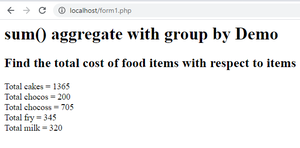My Personal Notes arrow_drop_up
Related Articles